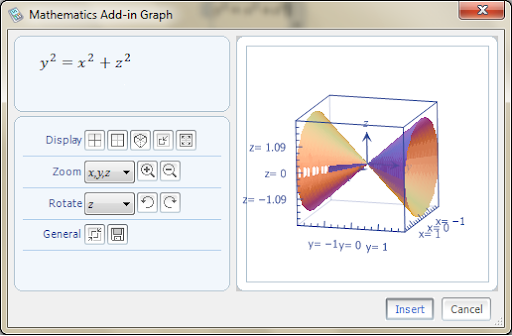# Microsoft Mathematics Add-In for Word and OneNote

Microsoft Mathematics Add-in for Word and OneNote is a set of tools that you can use to perform mathematical operations on expressions or equations in your Word documents and OneNote notebooks. The Mathematics add-in, which works with Microsoft Word 2010, Microsoft Office Word 2007, and Microsoft OneNote 2010, makes it easy to plot graphs in 2D and 3D, calculate numerical results, solve equations or inequalities, and simplify algebraic expressions.

The add-in provides an extensive collection of mathematical symbols and structures to display clearly formatted mathematical expressions. It automatically formats your input into mathematical notation. For example, if you enter int x^2 dx, it is automatically reformatted as ∫ x²dx. You can also quickly insert commonly used expressions and math structures by using the Equation gallery.The add-in uses dynamic 3D graphs and charts to help educators illustrate complex math problems and concepts. From algebra and pre-calculus to physics and statistics, teachers and students can unravel equations and visualize formulas through 2-D and 3-D graphs. It can plot functions, calculate numerical results, and dynamically solve for "x".

The Microsoft Mathematics Add-in, can be used to perform the following tasks:

• Compute standard mathematical functions, such as roots and logarithms
• Compute trigonometric functions, such as sine and cosine
• Find derivatives and integrals, limits, and sums and products of series
• Perform matrix operations, such as inverses, addition, and multiplication
• Perform operations on complex numbers
• Plot 2-D graphs in Cartesian and polar coordinates, and 3-D graphs in Cartesian, cylindrical, and spherical coordinates
• Solve equations and inequalities
• Calculate statistical functions, such as mode and variance, on lists of numbers
• Factor polynomials or integers
• Simplify or expand algebraic expressions

Also see, Microsoft Chemistry add-in for Microsoft Word

1.